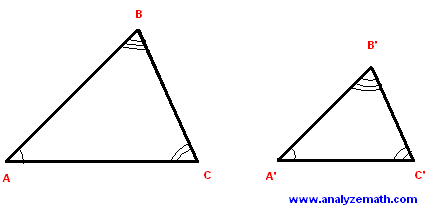### Popular Pages# Similar Triangles Examples

Definitions and theorems related to similar triangles are discussed using examples. Also Problems on similar triangles may be found in this site.

## Definition

Two triangles ABC and A'B'C' are similar if the three angles of the first triangle are congruent to the corresponding three angles of the second triangle and the lengths of their corresponding sides are proportional as follows.
AB / A'B' = BC / B'C' = CA / C'A'## Theorem

### Angle-Angle (AA) Similarity

If two angles in a triangle are congruent to the two corresponding angles in a second triangle, then the two triangles are similar.

### Example 1

Let ABC be a triangle and A'C' a segment parallel to AC. What can you say about triangles ABC and A'BC'? Explain your answer.Solution to Example 1:

• Since A'C' is parallel to AC, angles BA'C' and BAC are congruent. Also angles BC'A' and BCA are congruent. Since the two triangles have two corresponding congruent angles, they are similar.

## Theorem

### Side-Side-Side (SSS) Similarity

If the three sides of a triangle are proportional to the corresponding sides of a second triangle, then the triangles are similar.

### Example 2

Let the vertices of triangles ABC and PQR defined by the coordinates: A(-2,0), B(0,4), C(2,0), P(-1,1), Q(0,3), and R(1,1). Show that the two triangles are similar.

Solution to Example 2

• Let us first plot the vertices and draw the triangles.• Since we know the coordinates of the vertices, we can find the length of the sides of the two triangles.
AB = sqrt [ 4 2 + 2 2 ] = 2 sqrt(5)
BC = sqrt [ (-4) 2 + 2 2 ] = 2 sqrt(5)
CA = sqrt [ 4 2 ] = 4
PQ = sqrt [ 2 2 + 1 2 ] = sqrt(5)
QR = sqrt [ (-2) 2 + 1 2 ] = sqrt(5)
RP = sqrt [ 2 2 ] = 2
• We now calculate the ratios of the lengths of the corresponding sides.
AB / PQ = 2 , BC / QR = 2 and CA / RP = 2
• We can now write.
AB / PQ = BC / QR = CA / RP = 2
• The lengths of the corresponding sides are proportional and therefore the two triangles are similar.

## Theorem

### Side-Angle-Side (SAS) Similarity

If an angle of a triangle is congruent to the corresponding angle of a second triangle, and the lengths of the two sides including the angle in one triangle are proportional to the lengths of the corresponding two sides in the second triangle, then the two triangles are similar.

### Example 3

Show that triangles ABC and A'BC', in the figure below, are similar.Solution to Example 3:

• Angles ABC and A'BC' are congruent.
• Since the lengths of the sides including the congruent angles are given, let us calculate the ratios of the lengths of the corresponding sides.
BA / BA' = 10 / 4 = 5 / 2
BC / BC' = 5 / 2
• The two triangles have two sides whose lengths are proportional and a congruent angle included between the two sides. The two triangles are similar.

## More References and Links to Geometry Problems

Geometry Tutorials, Problems and Interactive Applets.